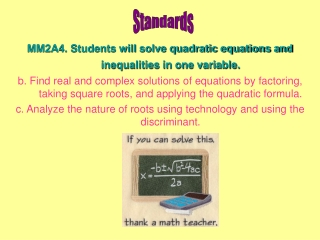DownloadDownload PresentationMM2A4. Students will solve quadratic equations and inequalities in one variable.

# MM2A4. Students will solve quadratic equations and inequalities in one variable.

Télécharger la présentation## MM2A4. Students will solve quadratic equations and inequalities in one variable.

- - - - - - - - - - - - - - - - - - - - - - - - - - - E N D - - - - - - - - - - - - - - - - - - - - - - - - - - -
##### Presentation Transcript

1. Standards MM2A4. Students will solve quadratic equations and inequalities in one variable. b. Find real and complex solutions of equations by factoring, taking square roots, and applying the quadratic formula. c. Analyze the nature of roots using technology and using the discriminant.

2. MM2A4bb. Find real and complex solutions of equations by factoring, taking square roots and applying the quadratic formula.

3. MM2A4bb. Find real and complex solutions of equations by factoring, taking square roots and applying the quadratic formula.

4. MM2A4bb. Find real and complex solutions of equations by factoring, taking square roots and applying the quadratic formula.

5. MM2A4bb. Find real and complex solutions of equations by factoring, taking square roots and applying the quadratic formula. Solve. x2 – 5x = 4 How many real solutions does this quadratic equation have? How many imaginary solutions does this quadratic equation have?

6. MM2A4bb. Find real and complex solutions of equations by factoring, taking square roots and applying the quadratic formula. Solve. 4x2 + 10x = -10x - 25 How many real solutions does this quadratic equation have? How many imaginary solutions does this quadratic equation have?

7. MM2A4bb. Find real and complex solutions of equations by factoring, taking square roots and applying the quadratic formula. Solve. x2 – 6x = -10 How many real solutions does this quadratic equation have? How many imaginary solutions does this quadratic equation have?

8. MM2A4bb. Find real and complex solutions of equations by factoring, taking square roots and applying the quadratic formula. The solutions to the quadratic equation are called the ROOTS of the equation

9. MM2A4bb. Find real and complex solutions of equations by factoring, taking square roots and applying the quadratic formula. You Try! Indicate whether the equation has two real roots, one real root, or two imaginary roots

10. MM2A4bb. Find real and complex solutions of equations by factoring, taking square roots and applying the quadratic formula. Which part of the quadratic formula determines the nature of the roots? This is called the DISCRIMINANT!

11. MM2A4cc. Analyze the nature of roots using technology and using the discriminant. Discriminant: b2 - 4ac • When the discriminant is ___________ there are 2 real roots. • When the discriminant is ___________ there is 1 real root. • When the discriminant is ___________ there are 2 imaginary roots.

12. MM2A4cc. Analyze the nature of roots using technology and using the discriminant.

13. MM2A4cc. Analyze the nature of roots using technology and using the discriminant. You Try!

14. MM2A4. Students will solve quadratic equations and inequalities in one variable.b. Find real and complex solutions of equations by factoring, taking square roots, and applying the quadratic formula. c. Analyze the nature of roots using technology and using the discriminant. Homework: pg. 93: 2 – 14 even pg. 94: 2 – 18 even WRITE PROBLEMS and SHOW WORK for credit!!